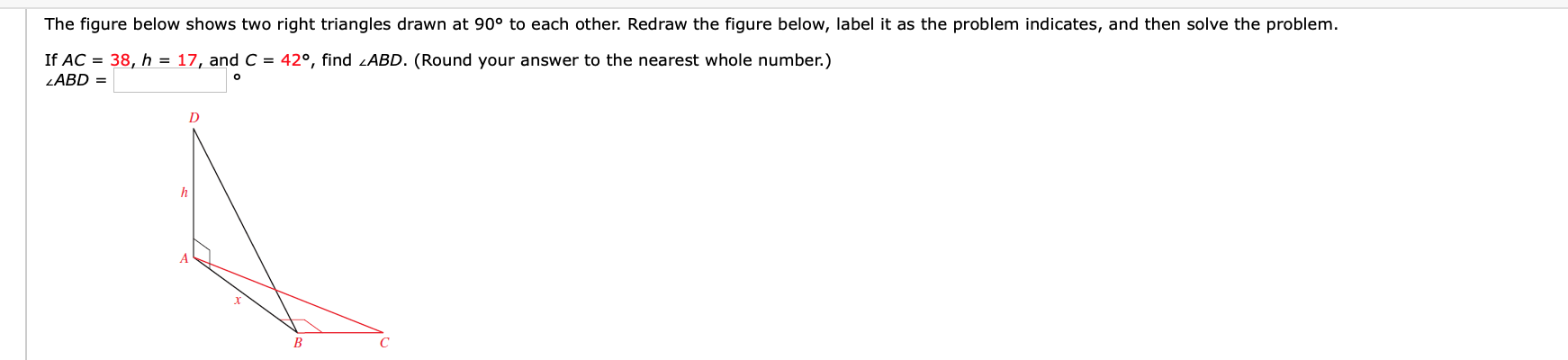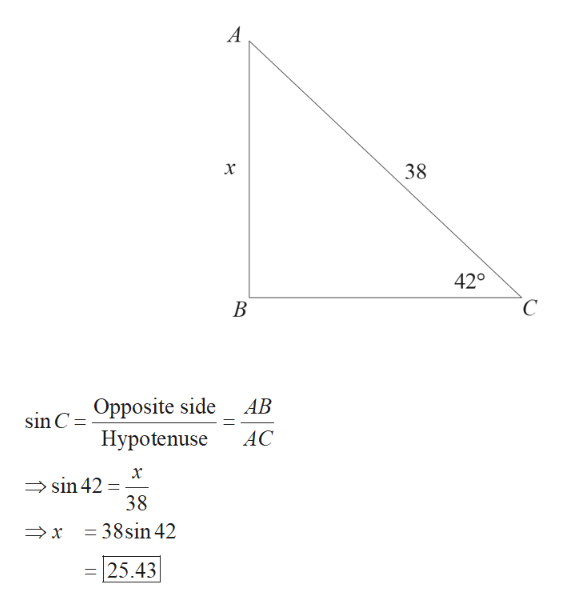# The figure below shows two right triangles drawn at 90° to each other. Redraw the figure below, label it as the problem indicates, and then solve the problemIf AC 38, h = 17, and C = 42°, find /ABD. (Round your answer to the nearest whole number.)LABD =hC

Question
1 viewshelp_outlineImage TranscriptioncloseThe figure below shows two right triangles drawn at 90° to each other. Redraw the figure below, label it as the problem indicates, and then solve the problem If AC 38, h = 17, and C = 42°, find /ABD. (Round your answer to the nearest whole number.) LABD = h C fullscreen
check_circle

Step 1

Redraw the triangle ABC and find the ...help_outlineImage TranscriptioncloseА 38 х 420 С В AB sin C-Opposite side Нурotenuse AC х sin 42 38 x =38sin 42 25.43 fullscreen

### Want to see the full answer?

See Solution

#### Want to see this answer and more?

Solutions are written by subject experts who are available 24/7. Questions are typically answered within 1 hour.*

See Solution
*Response times may vary by subject and question.
Tagged in

### Trigonometric Ratios